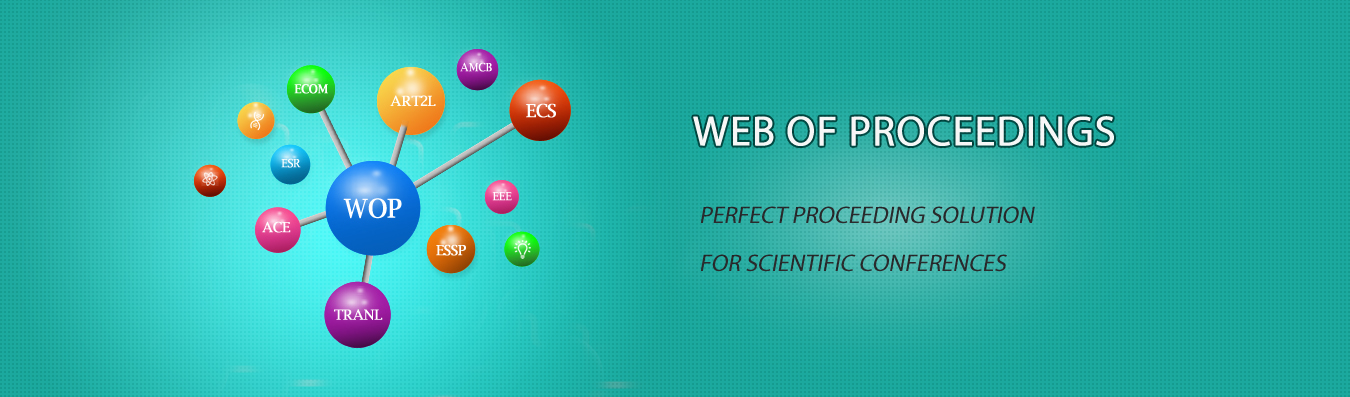The best way to conference proceedings by Francis Academic Press### Nonlinear Non Gaussian Kalman Filtering Algorithm Based on Gauss and 7th Order Volume

DOI: 10.25236/cseem.2020.156

Liu Zhengrong

Liu Zhengrong

#### Abstract

In the irregular Gaussian filter, the average quadratic root volume Kalman filter (sckf) has no filtering effect. In order to solve the problem of sckf and Gauss filtering, a new algorithm of Gauss root's average square volume Kalman filter is proposed. The algorithm uses Gauss type and formal type approximate non Gauss probability density, sckf as sub filter, updates the time and measurement of each Gaussian component, and effectively solves the problem of non-linear non Gaussian filtering. The simulation results show that the estimation accuracy of Gaussian filter and RMS volume Kalman filter is higher than that of particle filter, Gauss filter and extended Kalman filter. Nonlinear and non Gauss filtering algorithms for balancing tracking accuracy and real-time performance.

#### Keywords

Non-linear Non Gaussian, Gaussian Sum Filtering, Seven Order Volume Kalman Filtering Algorithm